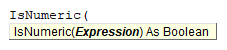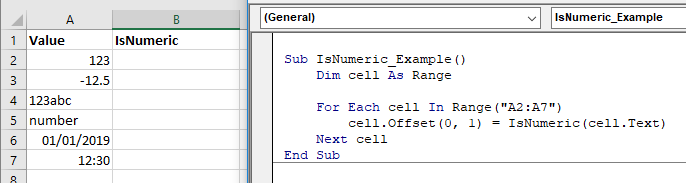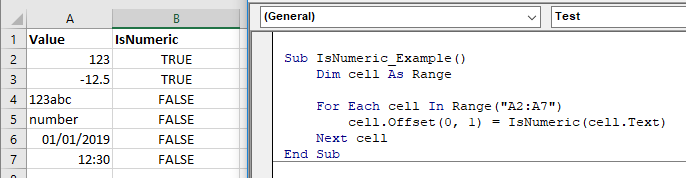# VBA IsNumeric Function

## IsNumeric Description

Used to check for a numeric value.

## Simple IsNumeric Examples

``MsgBox IsNumeric("123")``

Result: True

``MsgBox IsNumeric("123ABC")``

Result: False

## IsNumeric Syntax

In the VBA Editor, you can type  “IsNumeric(” to see the syntax for the IsNumeric Function:The IsNull function contains an argument:

Expression: An expression that will be evaluated.

## Examples of Excel VBA IsNumeric Function

``MsgBox IsNumeric("Number")``

Result: False

``MsgBox IsNumeric("12.34")``

Result: True

``MsgBox IsNumeric("12:00")``

Result: False

To check the cells on Excel Sheet, you can use the following example.``````Sub IsNumeric_Example()
Dim cell As Range

For Each cell In Range("A2:A7")
cell.Offset(0, 1) = IsNumeric(cell.Text)
Next cell
End Sub``````Stop searching for VBA code online. Learn more about AutoMacro - A VBA Code Builder that allows beginners to code procedures from scratch with minimal coding knowledge and with many time-saving features for all users!